# Themes, topics - math word problems

1. Drive to NJ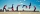Ed drove to New Jersey at 30mph. He drove back home in 3 hours at 50 mph. How many hours did it take Ed to drive to New Jersey?
2. Long bridge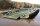Roman walked on the bridge. When he heard the whistle, he turned and saw running Kamil at the beginning of the bridge. If he went to him, they would meet in the middle of the bridge. Roman, however, rushed and so did not want to waste time returning 150m.
3. Accelerated motion - mechanicsThe delivery truck with a total weight of 3.6 t accelerates from 76km/h to 130km/h in the 0.286 km long way. How much was the force needed to achieve this acceleration?
4. Two math problems1) The sum of twice a number and -6 is nine more than the opposite of that number. Find the number. 2) A collection of 27 coins, all nickels, and dimes, is worth \$2.10. How many of each coin are there? The dime, in United States usage, is a ten-cent coi
5. Working aloneTom and Chandri are doing household chores. Chandri can do the work twice as fast as Tom. If they work together, they can finish the work in 5 hours. How long does it take Tom working alone to do the same work?
6. Intersect and conjuctionLet U={1,2,3,4,5,6} A={1,3,5} B={2,4,6} C={3,6} Find the following. 1. )AUB 2. )A'UB'
7. Three tributaries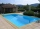It is possible to fill the pool with three tributaries. The first would take 12 hours, the second 15 hours, and the third 20 hours. The day before the summer season began, the manager opened all three tributaries simultaneously. How long did it take to fi
8. Two citiesCities A and B are 200 km away. At 7 o'clock from city A, the car started at an average speed of 80 km/h, and from B at 45 min later the motorcycle is started at an average speed of 120 km/h. How long will they meet and at what distance from the point A i
9. Temperature differenceThe highest temperature of Libya was recorded 58 degree Celsius and the lowest was recorded -88 degree Celsius, what is the temperature difference?
10. Six workersSix workers planned to repair the roof in 5 days. After two days, two workers get sick. How long will the remaining workers complete the roof at the same rate of work?
11. Four painters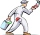The company sent four painters to paint the school. They should be done in 12 days. After three days, one painter got ill. How long will the remaining painters paint the school?
12. Assembly parts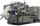Nine machines produce 1,800 parts on nine machines. How many hours will it produce 2 100 parts on seven such machines?
13. Seven workers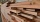Seven workers clear the glade in 22 hours. How many workers would need to be done in 8 hours?
14. IcebergWhat is the surface area of 50 cm iceberg (in the shape of a cuboid) that can carry a man with luggage with a total weight of 120 kg?
15. Sphere floatingWill float a hollow iron ball with an outer diameter d1 = 20cm and an inside diameter d2 = 19cm in the water? The iron density is 7.8 g/cm 3. (Instructions: Calculate the average sphere density and compare with the density of the water. )
16. Tourist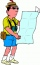A tourist walked an average speed of 3.5 km/h route in 6 hours. Calculate how many hours he would have passed at an average speed of 5.5 km/h.
17. Geometric plan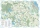At what scale the building plan if one side of the building is 45m long and 12mm long on a plan?
18. Avg speed of flight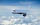The students vice adventure had a 2,367 km flight. If they travel time was 2 hours and 56 minutes, what was their average speed in kilometres per hour?
19. Speed of carIn 2 hours 40 mins, a car travels 100km. At what speed is the car traveling?
20. Friction coefficientWhat is the weight of a car when it moves on a horizontal road at a speed of v = 50 km/h at engine power P = 7 kW? The friction coefficient is 0.07

Do you have an interesting mathematical word problem that you can't solve it? Submit math problem, and we can try to solve it.

We will send a solution to your e-mail address. Solved examples are also published here. Please enter the e-mail correctly and check whether you don't have a full mailbox.

Please do not submit problems from current active competitions such as Mathematical Olympiad, correspondence seminars etc...